Join the forum, it's quick and easy

Would you like to react to this message? Create an account in a few clicks or log in to continue.

# [討論] LSP檔疑問~!

2 posters##[討論] LSP檔疑問~!

(defun c:Spline2Circle()
(SETVAR "CMDECHO" 0)
(COMMAND "UNDO" "BE")
(SETQ OLDOSMODE (GETVAR "OSMODE"))
(SETQ OLDLAYEAR (GETVAR "CLAYER"))
(SETVAR "OSMODE" 0)
;(setq FTT (getint "\n 輸入圓直徑大小: "))
(princ "請選預替換的雲行線 ")
(setq en (ssget '((0 . "spline"))))
(setq ppp (sslength en))
(setq qqq 0)
(repeat ppp
(setq ggg (ssname en qqq))
;(command "-color" 1)
(command "LAYER" "M" "CIRCLE" "C" 1 "CIRCLE" "")
(setq hhh (entget ggg))
(setq PT (MEMBER (assoc 10 hhh) hhh))
(setq pt1 (cdr (CAR PT)))
(setq pt2 (cdr (NTH 8 PT)))
;(command "circle" "2p" PT1 (polar pt1 (* pi 1.5) FTT))
(command "circle" "2p" PT1 PT2)
(setq qqq (+ qqq 1)))
(command "erase" en "")
;(command "-color" "bylayer")
(princ (strcat "\n共有 " (itoa ppp) " 個雲行線物件替換成圓物件...."))
(SETVAR "OSMODE" OLDOSMODE)
(SETVAR "CLAYER" OLDLAYEAR)
(COMMAND "UNDO" "E")
(prin1)
)
↑蘋果爸之前給的檔案~相當快速好用~想請問一下蘋果爸 如果要把雲行線改成橢圓轉正圓 需要改哪個地方 我找LSP黨找不到解說x26811199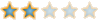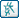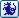##回復： [討論] LSP檔疑問~!

`(defun c:ellipse2Circle()  (SETVAR "CMDECHO" 0)  (COMMAND "UNDO" "BE")  (SETQ OLDOSMODE (GETVAR "OSMODE"))  (SETQ OLDLAYEAR (GETVAR "CLAYER"))  (SETVAR "OSMODE" 0)  ;(setq FTT (getint "\n 輸入圓直徑大小: "))  (princ "請選預替換的橢圓 ")  (setq en (ssget '((0 . "ellipse"))))  (setq ppp (sslength en))  (setq qqq 0)  (repeat ppp    (setq ggg (ssname en qqq))    ;(command "-color" 1)    (command "LAYER" "M" "CIRCLE" "C" 1 "CIRCLE" "")    (setq hhh (entget ggg))    (setq PT (MEMBER (assoc 10 hhh) hhh))    (setq PT2 (MEMBER (assoc 11 hhh) hhh))    (setq pt1 (cdr (CAR PT)))    (setq pt2 (cdr (CAR PT2)))    ;(command "circle" "2p" PT1 (polar pt1 (* pi 1.5) FTT))    (command "circle" PT1 PT2)    (setq qqq (+ qqq 1)))  (command "erase" en "")  ;(command "-color" "bylayer")  (princ (strcat "\n共有 " (itoa ppp) " 個橢圓物件替換成圓物件...."))  (SETVAR "OSMODE" OLDOSMODE)  (SETVAR "CLAYER" OLDLAYEAR)  (COMMAND "UNDO" "E")  (prin1)  )`

(setq PT (MEMBER (assoc 10 hhh) hhh))
(setq pt1 (cdr (CAR PT)))
(setq pt2 (cdr (NTH 8 PT)))

(setq PT (MEMBER (assoc 10 hhh) hhh))
(setq PT2 (MEMBER (assoc 11 hhh) hhh))
(setq pt1 (cdr (CAR PT)))
(setq pt2 (cdr (CAR PT2)))

(command "circle" "2p" PT1 PT2)

(command "circle" PT1 PT2)

leoneriol 在 2011-11-10, 13:00 作了第 1 次修改 (原因 : 程式碼小修正)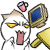leoneriol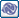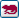##回復： [討論] LSP檔疑問~!x26811199##回復： [討論] LSP檔疑問~!

12MM~>181MM
15MM~>147MMx26811199##回復： [討論] LSP檔疑問~!

`(defun c:ellipse2Circle()  (SETQ OLDOSMODE (GETVAR "OSMODE"))  (SETQ OLDLAYEAR (GETVAR "CLAYER"))  (SETVAR "CMDECHO" 0)  (SETVAR "OSMODE" 0)  (princ "請選預替換的橢圓 ")  (setq en (ssget '((0 . "ellipse"))))  (setq n (sslength en))  (setq i 0)  (command "LAYER" "M" "CIRCLE" "C" 1 "CIRCLE" "")  (repeat n    (setq enidate (entget (ssname en i)))    (setq PT1 (cdr (assoc 10 enidate)))    (setq PT2 (cdr (assoc 11 enidate)))    (setq PT3 (polar Pt1 0 (distance '(0 0) pt2)))    (command "circle" PT1 PT3)    (setq i (+ i 1)))  (command "erase" en "")  (princ (strcat "\n共有 " (itoa n) " 個橢圓物件替換成圓物件...."))  (SETVAR "OSMODE" OLDOSMODE)  (SETVAR "CLAYER" OLDLAYEAR)  (prin1)  )`leoneriol